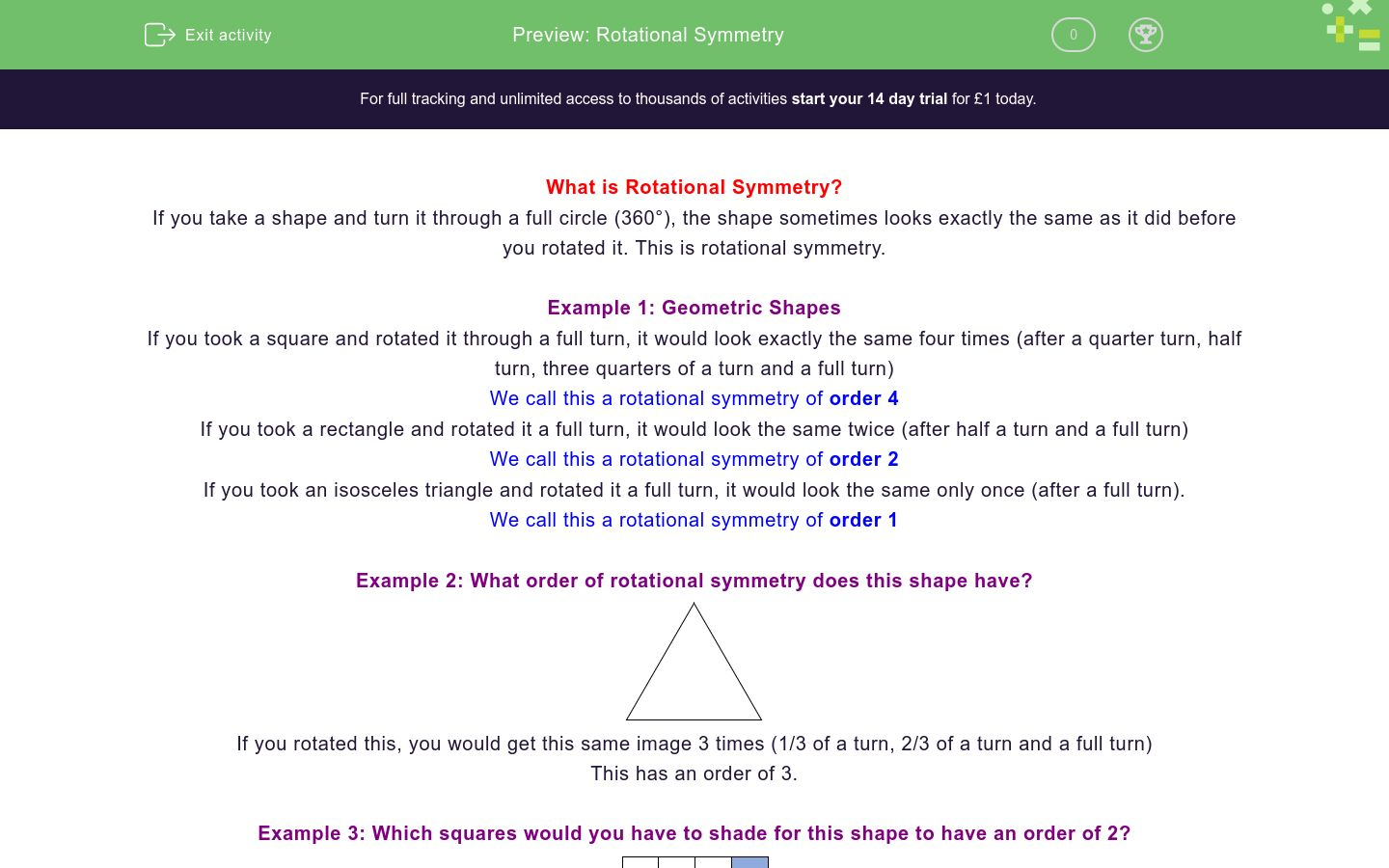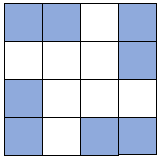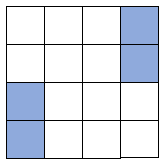# Rotational Symmetry

In this worksheet, students practise using orders of rotational symmetry.Key stage:  KS 4

GCSE Subjects:   Maths

GCSE Boards:   AQA, Pearson Edexcel, Eduqas, OCR

Curriculum topic:   Geometry and Measures, Congruence and Similarity

Curriculum subtopic:   Properties and Constructions, Plane Isometric Transformations

Difficulty level:### QUESTION 1 of 10

What is Rotational Symmetry?

If you take a shape and turn it through a full circle (360°), the shape sometimes looks exactly the same as it did before you rotated it. This is rotational symmetry.

Example 1: Geometric Shapes

If you took a square and rotated it through a full turn, it would look exactly the same four times (after a quarter turn, half turn, three quarters of a turn and a full turn)

We call this a rotational symmetry of order 4

If you took a rectangle and rotated it a full turn, it would look the same twice (after half a turn and a full turn)

We call this a rotational symmetry of order 2

If you took an isosceles triangle and rotated it a full turn, it would look the same only once (after a full turn).

We call this a rotational symmetry of order 1

Example 2: What order of rotational symmetry does this shape have?If you rotated this, you would get this same image 3 times (1/3 of a turn, 2/3 of a turn and a full turn)

This has an order of 3.

Example 3: Which squares would you have to shade for this shape to have an order of 2?Order 2 means it would look the same twice. If you divide the full turn (360°) by this you would get that it looks the same every 180°.

If we rotated the shape 180°, the shaded shapes would be in the bottom left corner.Example 3: Which squares would you have to shade for this shape to have an order of 4?Order 4 means it would look the same twice. If you divide the full turn (360°) by this you would get that it looks the same every 90°.

If we rotated the shape 90°, the shaded shapes would be in the bottom right corner.

If we rotated the shape another 90°, the shaded shapes would be in the bottom left corner.

If we rotated the shape another 90°, the shaded shapes would be in the top left corner.Rotational symmetry is where you take a shape and turn it through a full circle (360°)...

What order of rotational symmetry does a square have?

1

2

3

4

What order of rotational symmetry does a rectangle have?

2

4

Triangles have a different order of rotational symmetry depending on what type of triangle they are.

2

4

Match the order of rotational symmetry with the shape.

## Column B

Square
6
Equilateral Triangle
4
Regular Hexagon
10
Regular Decagon
3

What is the rotational symmetry of this shape?What is the rotational symmetry of this shape?Which of the squares (A-M) would you need to shade to get a rotational symmetry of order 2?A

B

C

D

E

F

G

H

I

J

K

L

M

Which of the squares (A-M) would you need to shade to get a rotational symmetry of order 4?A

B

C

D

E

F

G

H

I

J

K

L

M

What is the order of rotational symmetry of a regular polygon?

A

B

C

D

E

F

G

H

I

J

K

L

M

• Question 1

Rotational symmetry is where you take a shape and turn it through a full circle (360°)...

EDDIE SAYS
Its really important that you get this as the word is going to be used in the exam. We call it the order
• Question 2

What order of rotational symmetry does a square have?

4
EDDIE SAYS
A square is a regular shape. Regular shapes will always have the same order of rotational symmetry as their sides,
• Question 3

What order of rotational symmetry does a rectangle have?

2
EDDIE SAYS
It really common to say 4 for this. Try it, take a piece of paper and turn it 90°. Does it look the same as when you started?
• Question 4

Triangles have a different order of rotational symmetry depending on what type of triangle they are.

EDDIE SAYS
The equilateral triangle is a regular shape. What does this tell us about the order? The mistake a lot of people make here is to say that a scalene has an order of 0. No shape can have a rotational order of 0. All shapes will look exactly the same when you have turned them through a full circle. The lowest number you can have for an order of rotational symmetry is 1.
• Question 5

Match the order of rotational symmetry with the shape.

## Column B

Square
4
Equilateral Triangle
3
Regular Hexagon
6
Regular Decagon
10
EDDIE SAYS
Did you spot that these are all regular shapes? Remember the fact that a regular shape will have the same order of rotational symmetry as it does sides.
• Question 6

What is the rotational symmetry of this shape?2
two
EDDIE SAYS
If you turned this through a full circle, how many times would it look exactly like this?
• Question 7

What is the rotational symmetry of this shape?4
four
EDDIE SAYS
If you turned this through a full circle, how many times would it look exactly like this?
• Question 8

Which of the squares (A-M) would you need to shade to get a rotational symmetry of order 2?A
E
F
EDDIE SAYS
Remember that order 2 means it will look exactly the same is you rotate it through 180° Where would the three shaded squares be after you did that?
• Question 9

Which of the squares (A-M) would you need to shade to get a rotational symmetry of order 4?A
C
D
E
F
G
J
K
L
EDDIE SAYS
Remember that order 4 means it will look exactly the same is you rotate it through 90° three times Where would the three shaded squares be when you did that?
• Question 10

What is the order of rotational symmetry of a regular polygon?

EDDIE SAYS
Remember that a polygon is just a shape with straight-line sides (including triangles, quadrilaterals etc) Regular just means all the sides and internal angles are the same. What does this tell us about the order of rotational symmetry?
---- OR ----

Sign up for a £1 trial so you can track and measure your child's progress on this activity.

### What is EdPlace?

We're your National Curriculum aligned online education content provider helping each child succeed in English, maths and science from year 1 to GCSE. With an EdPlace account you’ll be able to track and measure progress, helping each child achieve their best. We build confidence and attainment by personalising each child’s learning at a level that suits them.

Get started